# # 单向链表

TIP

## # 链表和数组优缺点对比

### # 数组的缺点：

• 数组的创建通常需要申请一段连续的内存空间（一整块内存），并且大小是固定的
• 如果原数组不能满足容量需求时，需要扩容（一般是申请一个更大的数组，比如 2 倍，然后将原数组中的元素复制过去）
• 在数组的开头或中间位置插入数据的成本很高，需要进行大量元素的位移

### # 链表的优势：

• 内存空间不必是连续的，元素可以任意放置，可以充分利用计算机的内存，实现灵活的内存动态管理
• 链表不必在创建时就确定大小，并且大小可以无限地延伸下去
• 链表在插入和删除数据时，时间复杂度可以达到 O(1)，相对数组效率高很多

### # 链表的缺点：

• 链表访问任何一个位置的元素时，都需要从头开始访问（无法跳过第一个元素访问任何一个元素）
• 无法通过下标值直接访问元素，需要从头开始一个个访问，直到找到对应的元素

## # 基本特点

• 每个节点包含 item(data) 数据和 next 指针
• 链表中的最后一个节点指向 null

## # 结构图例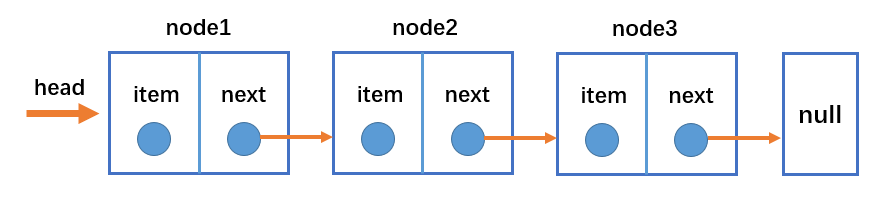## # 位置图例

position 的值一般表示 position 所指位置的下一个节点。当 position 的值与 index 的值相等时，比如 position = index = 1，那么它们都表示 Node2。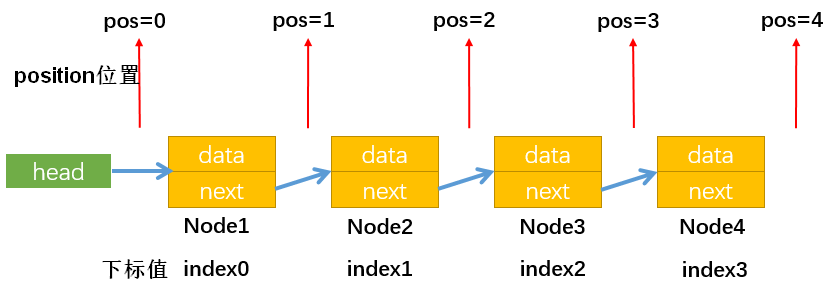## # 常用操作

• append(element)：向链表尾部添加一个新的项
• insert(position, element)：向链表的特定位置插入一个新的项
• get(position)：获取对应位置的元素
• indexOf(element)：返回元素在链表中的索引。如果链表中没有该元素就返回-1
• update(position, element)：修改某个位置的元素
• removeAt(position)：从链表的特定位置移除一项
• remove(element)：从链表中移除一项
• isEmpty()：如果链表中不包含任何元素，返回 true，如果链表长度大于 0，返回 false
• size()：返回链表包含的元素个数，与数组的 length 属性类似
• toString()：由于链表项使用了 Node 类，就需要重写继承自 JS 对象默认的 toString 方法，让其只输出元素的值

## # 代码实现

### # 0、创建单向链表类

``````// 封装链表类
// 封装一个内部类：节点类(data:数据)
function Node(data) {
this.data = data // 数据
this.next = null // 下一个指针引用
}

/* 属性 */
// 记录链表的长度，默认为0
this.length = 0
}
``````

### # 1、toString 方法

``````// 1 toString(): 转化字符串方法
// 1.1 定义变量
var resultString = ""

// 1.2 通过while循环获取一个一个节点
while (current) {
resultString += current.data + " "
// 拼接完一个节点数据之后，让current指向下一个节点
current = current.next
}

// 1.3 返回字符串
return resultString
}
``````

### # 2、append 方法

``````// 2. append(): 向尾部添加一个数据
// 2.1 创建新节点
var newNode = new Node(data)

// 2.2 判断添加的是否是第一个节点
if (this.length === 0) {
// 是第一个节点，直接指向新节点
} else {
// 不是第一个节点，让current先指向第一个节点
// 通过while循环查找，直到current.next为null停止循环，找到最后一个节点
while (current.next) {
current = current.next
}
// 最后节点的next指向新的节点
current.next = newNode
}

// 2.3 添加完新结点之后长度+1
this.length++
}
``````

• 通过 while 循环使 current 指向最后一个节点，最后通过 current.next = newNode，让最后一个节点指向新节点 newNode``````var link = new LinkList()

``````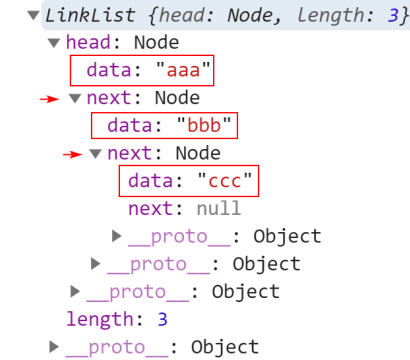### # 3、insert 方法

``````// 3. insert方法: 向特定位置插入一条新的数据
//理解positon的含义：position=0表示新节点插入后要成为第1个节点，position=2表示新节点插入后要成为第3个节点
if (position < 0 || position > this.length) {
return false
}
// 3.2 根据data创建新节点newNode
var newNode = new Node(data)

// 3.3 判断插入的位置是否是第一个
if (position === 0) {
// 情况1：插入位置position=0
// 让新节点指向第一个节点
} else {
// 情况2：插入位置position > 0(该情况包含position=length)
var index = 0
var previous = null

// 步骤1：通过while循环使变量current指向position位置的后一个节点(注意while循环的写法)
while (index++ < position) {
// 步骤2：让previous指向current当前指向的节点，然后current指向下一个节点
previous = current
current = current.next
}
// 步骤3：通过变量current，使newNode指向position位置的后一个节点
newNode.next = current
// 步骤4：通过变量previous，使position位置的前一个节点指向newNode
previous.next = newNode

/*
启示：
1.我们无法直接操作链表中的节点，但是可以通过变量指向这些节点，以此间接地操作节点(替身使者)；
比如current指向节点3，想要节点3指向节点4只需要：current.next = 4即可。
2.两个节点间是双向的，想要节点2的前一个节点为节点1，可以通过：1.next=2，来实现；
*/
}

// 3.4 新节点插入后要length+1
this.length += 1
return true
}
``````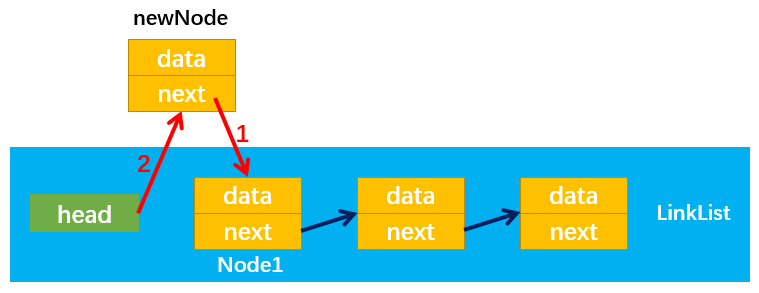• 首先定义两个变量 previous 和 current 分别指向需要插入位置 pos = X 的前一个节点 2 和后一个节点 1
• 然后，通过：newNode.next = current，改变指向 3
• 最后，通过：previous.next = newNode，改变指向 4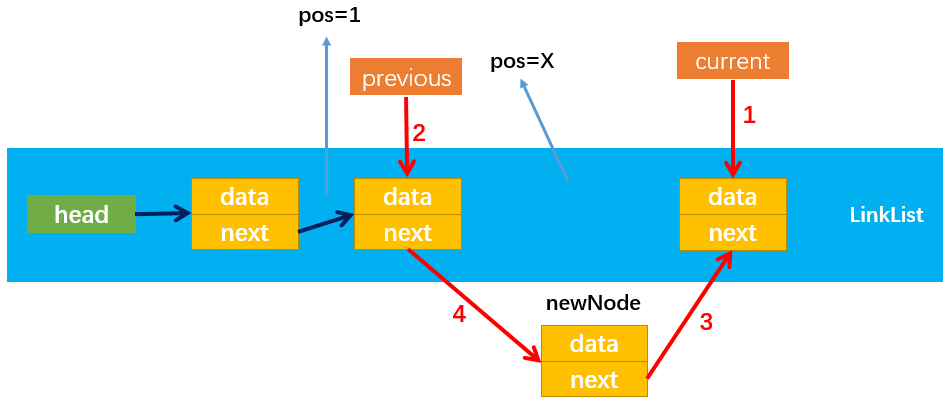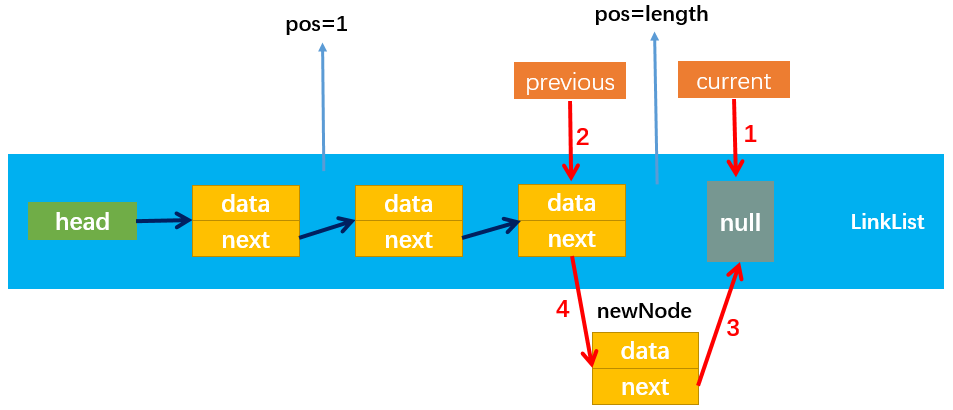``````// 测试insert
console.log(link.toString()) // 0a aaa bbb 3b ccc 5c
``````

### # 4、get 方法

``````// 4. get方法：获取对应位置的元素
// 4.1 越界判断：当position=length时，取到的是null，所以0=<position<length
if (position < 0 || position >= this.length) return null

// 4.2 获取指定的positon位置的后一个节点的data
var index = 0
// 通过while循环，直到index = position，此时curent表示position后一个节点
while (index++ < position) {
current = current.next
}
// 4.3 返回data数据
return current.data
}
``````

• get 方法的实现过程：以获取 position = 2 为例，如下图所示
• 首先使 current 指向第一个节点，此时 index=0
• 通过 while 循环使 current 循环指向下一个节点，注意循环终止的条件 index++ < position，即当 index=position 时停止循环，此时循环了 1 次，current 指向第二个节点(Node2)，最后通过 current.data 返回 Node2 节点的数据；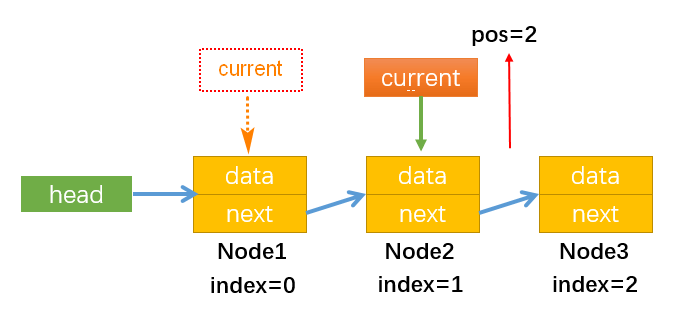``````// 已有：0a aaa bbb 3b ccc 5c
``````

### # 5、indexOf 方法

``````// 5. indexOf: 返回元素在列表中的索引，如果列表中没有该元素则返回-1
// 5.1 定义变量
var index = 0
// 5.2 开始查找: 只要current不指向null就一直循环
while (current) {
if (current.data === data) {
return index
}
current = current.next
index += 1
}
// 5.3 找到最后没有找到，返回-1
return -1
}
``````

• 使用变量 current 记录当前指向的节点，使用变量 index 记录当前节点的索引值（注意 index = node 数-1）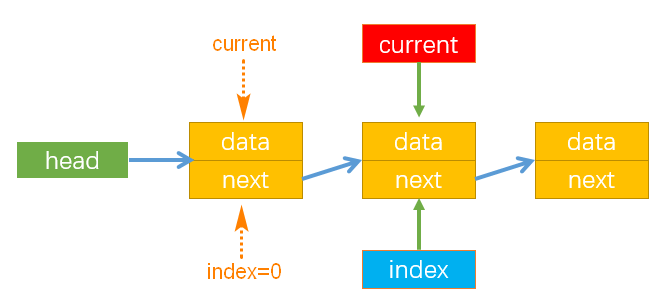``````// 已有：0a aaa bbb 3b ccc 5c
``````

### # 6、update 方法

``````// 6. update方法：修改某个位置的元素
// 6.1 越界判断: 因为被修改的节点不能为null，所以position不能等于length
if (position < 0 || position >= this.length) {
return false
}
// 6.2 查找正确的节点
let index = 0
while (index++ < position) {
current = current.next
}
// 6.3 将position位置的后一个节点的data修改成newData
current.data = newData
// 6.4 返回true表示修改成功
return true
}
``````

``````// 已有：0a aaa bbb 3b ccc 5c
console.log(link.toString()) // mmm aaa bbb nnn ccc 5c
``````

### # 7、removeAt 方法

``````// 7. removeAt: 从特定位置移除一项
// 7.1 越界判断：position不能等于length，因为等于length的节点为null
if (position < 0 || position >= this.length) {
return null
}
// 7.2 删除元素
if (position === 0) {
// 情况1：position = 0时(删除第一个节点)
} else {
// 情况2：position > 0时
var index = 0
var previous = null
while (index++ < position) {
previous = current
current = current.next
}
// 循环结束后，current指向position后一个节点，previous指向current前一个节点
// 再使前一个节点的next指向current的next即可
previous.next = current.next
}
// 7.3 length要减1
this.length -= 1
// 7.4 返回被删除节点的data，为此current定义在最上面
return current.data
}
``````

• 虽然 Node1 的 next 仍指向 Node2，但是没有引用指向 Node1，则 Node1 会被垃圾回收器自动回收，所以不用处理 Node1 指向 Node2 的引用 next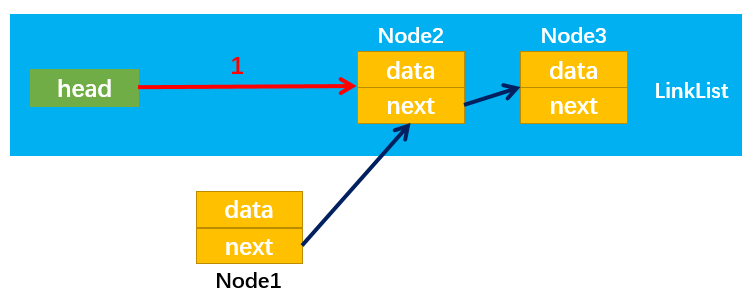• 比如 pos = 2 即移除第三个节点（Node3）
• 首先，定义两个变量 previous 和 curent 分别指向需要删除位置 pos = x 的前一个节点和后一个节点
• 然后，通过：previous.next = current.next，改变指向 1 即可
• 随后，没有引用指向 Node3，Node3 就会被自动回收，至此成功删除 Node3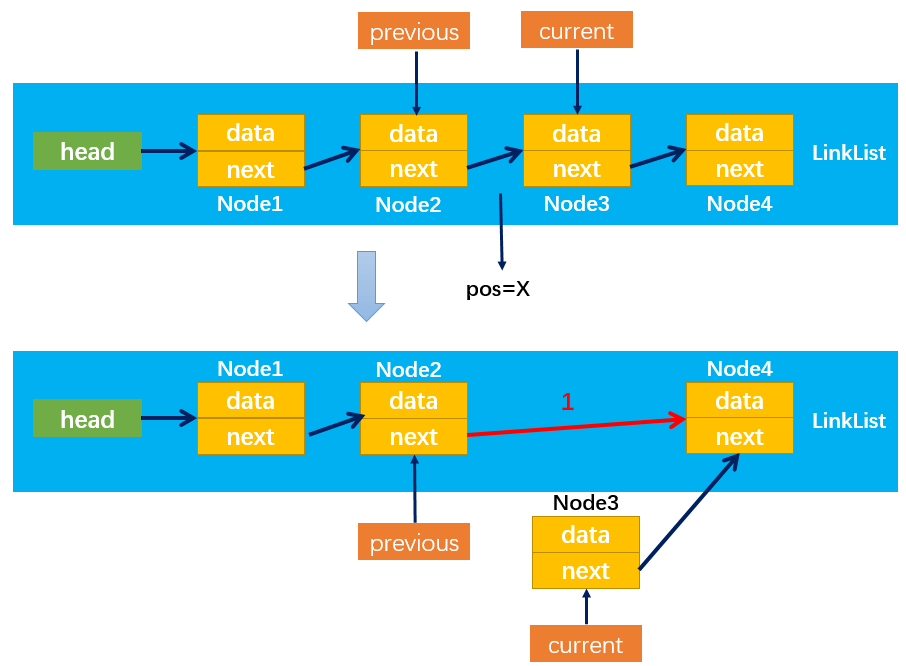``````// 已有：mmm aaa bbb nnn ccc 5c
// 已有：aaa bbb nnn ccc 5c
``````

### # 8、remove|isEmpty|size 方法

``````// 8. remove方法：从列表移除一项
// 8.1 获取data在列表中的位置
var position = this.indexOf(data)
// 8.2 根据位置信息删除节点
return this.removeAt(position)
}

// 9. isEmpty方法：为空返回true，不为空返回false
return this.length === 0
}

// 10. size方法：返回节点个数
return this.length
}
``````

``````// 已有：aaa bbb nnn 5c

// 已有：aaa bbb 5c
``````

### # 9、完整代码

``````// 封装链表类
// 封装一个内部类：节点类(data:数据)
function Node(data) {
this.data = data // 数据
this.next = null // 下一个指针引用
}

/* 属性 */
// 记录链表的长度，默认为0
this.length = 0

// 1 toString(): 转化字符串方法
// 1.1 定义变量
var resultString = ""

// 1.2 通过while循环获取一个一个节点
while (current) {
resultString += current.data + " "
// 拼接完一个节点数据之后，让current指向下一个节点
current = current.next
}

// 1.3 返回字符串
return resultString
}

// 2. append(): 添加方法
// 2.1 创建新节点
var newNode = new Node(data)

// 2.2 判断添加的是否是第一个节点
if (this.length === 0) {
// 是第一个节点，直接指向新节点
} else {
// 不是第一个节点，让current先指向第一个节点
// 通过while循环查找，直到current.next为null停止循环，找到最后一个节点
while (current.next) {
current = current.next
}
// 最后节点的next指向新的节点
current.next = newNode
}

// 2.3 添加完新结点之后长度+1
this.length++
}

// 3. insert方法: 向特定位置插入一条新的数据
//理解positon的含义：position=0表示新节点插入后要成为第1个节点，position=2表示新节点插入后要成为第3个节点
if (position < 0 || position > this.length) {
return false
}
// 3.2 根据data创建新节点newNode
var newNode = new Node(data)

// 3.3 判断插入的位置是否是第一个
if (position === 0) {
// 情况1：插入位置position=0
// 让新节点指向第一个节点
} else {
// 情况2：插入位置position > 0(该情况包含position=length)
var index = 0
var previous = null

// 步骤1：通过while循环使变量current指向position位置的后一个节点(注意while循环的写法)
while (index++ < position) {
// 步骤2：让previous指向current当前指向的节点，然后current指向下一个节点
previous = current
current = current.next
}
// 步骤3：通过变量current，使newNode指向position位置的后一个节点
newNode.next = current
// 步骤4：通过变量previous，使position位置的前一个节点指向newNode
previous.next = newNode

/*
启示：
1.我们无法直接操作链表中的节点，但是可以通过变量指向这些节点，以此间接地操作节点(替身使者)；
比如current指向节点3，想要节点3指向节点4只需要：current.next = 4即可。
2.两个节点间是双向的，想要节点2的前一个节点为节点1，可以通过：1.next=2，来实现；
*/
}

// 3.4 新节点插入后要length+1
this.length += 1
return true
}

// 4. get方法：获取对应位置的元素
// 4.1 越界判断：当position=length时，取到的是null，所以0=<position<length
if (position < 0 || position >= this.length) return null

// 4.2 获取指定的positon位置的后一个节点的data
var index = 0
// 通过while循环，直到index = position，此时curent表示position后一个节点
while (index++ < position) {
current = current.next
}
// 4.3 返回data数据
return current.data
}

// 5. indexOf: 返回元素在列表中的索引，如果列表中没有该元素则返回-1
// 5.1 定义变量
var index = 0
// 5.2 开始查找: 只要current不指向null就一直循环
while (current) {
if (current.data === data) {
return index
}
current = current.next
index += 1
}
// 5.3 找到最后没有找到，返回-1
return -1
}

// 6. update方法：修改某个位置的元素
// 6.1 越界判断: 因为被修改的节点不能为null，所以position不能等于length
if (position < 0 || position >= this.length) {
return false
}
// 6.2 查找正确的节点
let index = 0
while (index++ < position) {
current = current.next
}
// 6.3 将position位置的后一个节点的data修改成newData
current.data = newData
// 6.4 返回true表示修改成功
return true
}

// 7. removeAt: 从特定位置移除一项
// 7.1 越界判断：position不能等于length，因为等于length的节点为null
if (position < 0 || position >= this.length) {
return null
}
// 7.2 删除元素
if (position === 0) {
// 情况1：position = 0时(删除第一个节点)
} else {
// 情况2：position > 0时
var index = 0
var previous = null
while (index++ < position) {
previous = current
current = current.next
}
// 循环结束后，current指向position后一个节点，previous指向current前一个节点
// 再使前一个节点的next指向current的next即可
previous.next = current.next
}
// 7.3 length要减1
this.length -= 1
// 7.4 返回被删除节点的data，为此current定义在最上面
return current.data
}

// 8. remove方法：从列表移除一项
// 8.1 获取data在列表中的位置
var position = this.indexOf(data)
// 8.2 根据位置信息删除节点
return this.removeAt(position)
}

// 9. isEmpty方法：为空返回true，不为空返回false
return this.length === 0
}

// 10. size方法：返回节点个数
return this.length
}
}

// 测试insert
console.log(link.toString()) // 0a aaa bbb 3b ccc 5c

// 已有：0a aaa bbb 3b ccc 5c

// 已有：0a aaa bbb 3b ccc 5c

// 已有：0a aaa bbb 3b ccc 5c
console.log(link.toString()) // mmm aaa bbb nnn ccc 5c

// 已有：mmm aaa bbb nnn ccc 5c